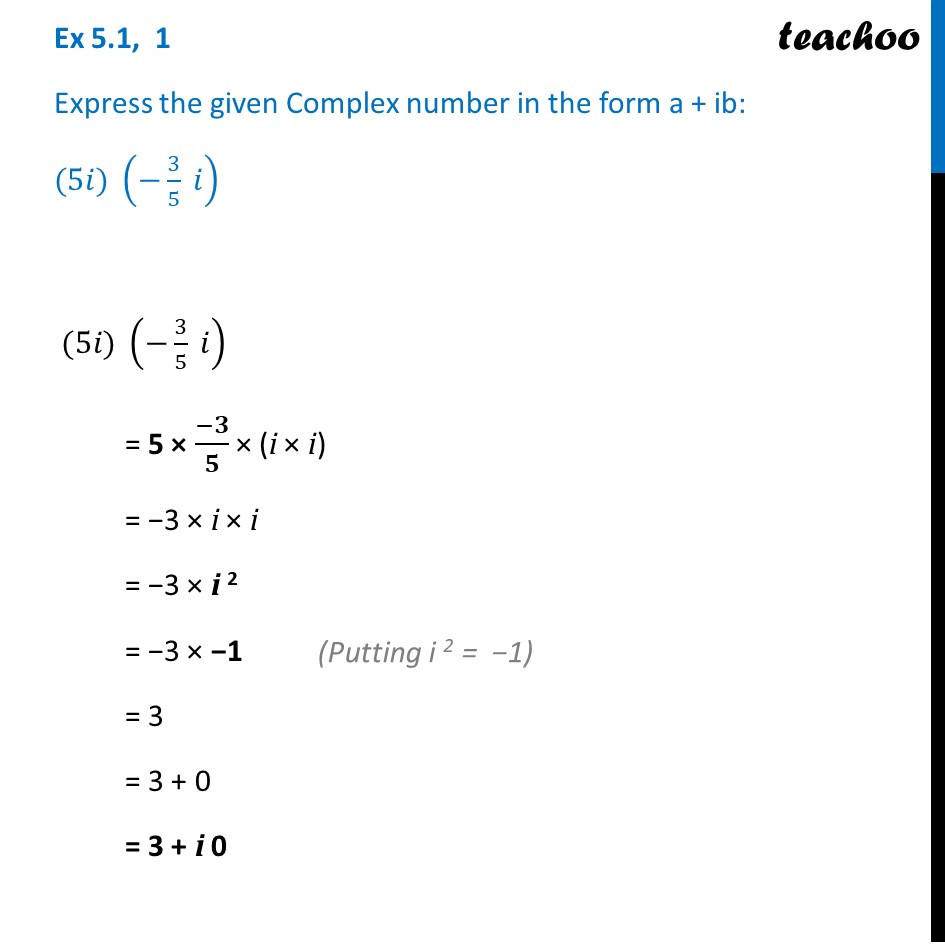Convert to a + ib form

Chapter 5 Class 11 Complex Numbers (Term 1)
Concept wise### Transcript

Ex 5.1, 1 Express the given Complex number in the form a + ib: (5𝑖) (−3/5 𝑖) (5𝑖) (−3/5 𝑖) = 5 × (−𝟑)/𝟓 × (i × i) = −3 × i × i = −3 × i 2 = −3 × −1 = 3 = 3 + 0 = 3 + i 0